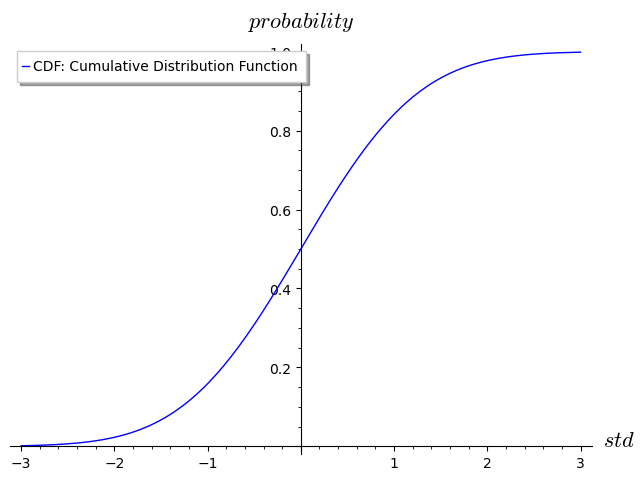# Black-Scholes formula

## Finance

Black-Scholes formula… maybe not as important and well-known as compounding interest-rate but still the most famous formula among options traders. Here are the formulas that you will find in financial books.

### Intuition

$d1 = \frac{\ln{\frac{\textcolor{blue}{S}}{\textcolor{cyan}{K}}} + (r + \frac{\textcolor{lime}{\sigma}^2}{2}) * \textcolor{magenta}{t}}{\textcolor{lime}{\sigma}*\sqrt{\textcolor{magenta}{t}}} \\ d2 = \frac{\ln{\frac{\textcolor{blue}{S}}{\textcolor{cyan}{K}}} + (r - \frac{\textcolor{lime}{\sigma}^2}{2}) * \textcolor{magenta}{t}}{\textcolor{lime}{\sigma}*\sqrt{\textcolor{magenta}{t}}}$

$\textcolor{green} {C} = N(d1) * \textcolor{blue}{S} - N(d2) * \textcolor{cyan}{K} * e^{-r*\textcolor{magenta}{t}}$

$\textcolor{red} {P} = N(-d2) * \textcolor{cyan}{K} * e^{-r*\textcolor{magenta}{t}} - N(-d1) * \textcolor{blue}{S}$

where:

• C - price of an European Call options
• P - price of an European Put options
• N - cumulative distribution function (CDF)
• S - Spot price of the underlying asset
• K - striKe price of option
• t - time to expiraTion (in years)
• σ - sigma - standard deviation of log returns (volatility)
• σ² - squared deviation (variance)

Scary? not really… let's put d1 and d2 terms aside for now and try to understand the call/put price formulas.

We know the discounted interest-rate formula, details and proof in this blog post.

$\color{blue} D = C * e^{-r*t}$

where

• C - the compounding value (forward/future price) after t time and D is present value (current price); or put the it other way around, D is the discounted value of C.
• D - present value (discounted value) of the strike price K

What about N( ) thing? First thing first, let's have a look at Gaussian distribution (also called normal distribution) with 1 standard deviation (σ = 1) and 0 mean (μ = 0)

  G = RealDistribution('gaussian', 1)
  PDF = lambda x: G.distribution_function(x)
plot(PDF, (x, -3, 3), legend_label='PDF: Probability Density Function', axes_labels=['$std$', '$gradient$'])Since PDF is probability density function we are dealing with areas of probabilities, in simple terms, the area to the left of the mean has 50% probability.

  CDF = lambda x: G.cum_distribution_function(x)
plot(CDF, (x, -3, 3), legend_label='CDF: Cumulative Distribution Function', axes_labels=['$std$', '$probability$'])CDF is called cumulative distribution function which is the probability of a random variable X to be less than a given point x.

Without going into details, the relation between the two is that, we can integrate the density function and find the cumulative function

$\int_{-\infty}^x PDF(x) \, \mathrm{d}x = CDF(x)$

and vice-versa, we differentiate the cumulative function and find the density function.

$\frac{\mathrm{d} \, CDF(x)}{\mathrm{d}x} = PDF(x)$

Finally, since our N( ) function is a CDF it means that it is 0 < N( ) < 1, in other words it is a weight (a scaling factor).

With this new knowledge, let's change the call/put price formulas to:

$\textcolor{green} {C} = N(d1) * \textcolor{blue}{S} - N(d2) * \textcolor{cyan}{D}$

$\textcolor{red} {P} = N(-d2) * \textcolor{cyan}{D} - N(-d1) * \textcolor{blue}{S}$

In conclusion:

the price for a CALL option can be viewed as:

• profit/loss amount: a scaling factor times the underlying price at expiry
• minus
• amount that we pay: scaling factor times discounted value of the strike price now

and a PUT options as:

• amount that we pay - scaling factor times discounted value of the strike price now
• minus
• profit/loss amount - scaling factor times the underlying spot price at expiration

### Implementation

In Python/Sagemath it looks like this:

  from math import log, sqrt, pi, exp

def d1(s, k, t, r, iv):
return(log(s/k) + (r+iv**2/2)*t) / iv*sqrt(t)
def d2(s, k, t, r, iv):
return d1(s,k,t,r,iv) - iv*sqrt(t)

def D(k, r, t):
return k * exp(-r*t)
def N(d):
return CDF(d)

def call_price(s, k, t, r, iv):
return N(d1(s,k,t,r,iv)) * s - N(d2(s,k,t,r,iv)) * D(k,r,t)
def put_price(s, k, t, r, iv):
return N(-d2(s,k,t,r,iv)) * D(k,r,t) - N(-d1(s,k,t,r,iv)) * s

Call price:

  s = 1330
k = 1280
t = (10 + 17/24) / 365
r = 0.01
iv = 1.34
print(call_price(s, k, t, r, iv))
141.6089647684081


Put price:

  s = 33760
k = 34000
t = (10 + 17/24) / 365
r = 0.01
iv = 1.10
print(put_price(s, k, t, r, iv))
2655.0941969718187


### Implied volatility

We can do it the other way around as well and recursively find the implied volatility for a given price.

Call IV:

  def call_iv(p, iv=1.30, step=0.01):
ip = call_price(s, k, t, r, iv)
if ip > p:
return iv;
else:
return call_iv(p, iv + step)

print(call_iv(141))
1.34000000000000


Put IV:

  def put_iv(p, iv=1.00, step=0.01):
ip = put_price(s, k, t, r, iv)
if ip > p:
return iv;
else:
return put_iv(p, iv + step)

print(put_iv(2660))
1.11000000000000